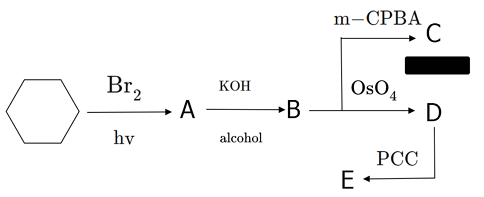# Oxygen - Carbon Bond Counter

Chemistry Level 2Starting with cyclohexane, proceed through the series of reactions shown above. For products $C$, $D$, and $E$; count the total number of $C-O$ (carbon to oxygen) bonds in each molecule. In the image above, m-CPBA stands for meta-chloroperoxybenzoic acid and PCC stands for pyridinium chlorochromate.

You answer should be the sum of the $C-O$ bonds in products $C$, $D$, and $E$.

Note: Be careful of certain groups; for example, a ketone will count as two $C-O$ bonds. Same is true for epoxides. And of course, carboxylic acids will contain three.

David's Organic Chemistry Set

David's Physical Chemistry Set

×